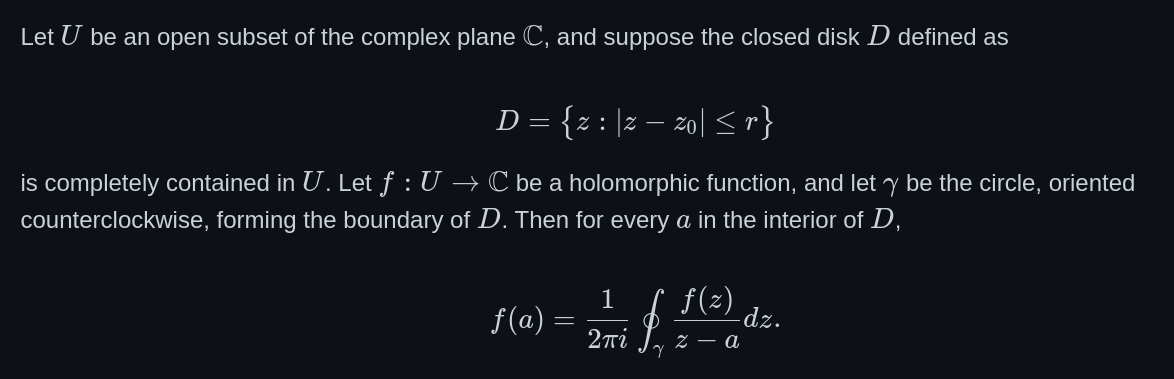Finally! On May 19, 2022, GitHub released one of its most requested features: Math support on Markdown! Many had been anticipating this for years, including myself, so much in fact that I wrote a browser extension to get there early. (xhub, it can do a few other things so check it out.)

The syntax GitHub has chosen is the familar $-based TeX syntax. Inline math:$a+b$Display math: $$a + b$$  I’m pointing out below why this may be a problem. ## The Good The best thing is that something is finally there. This #### Cauchy's Theorem Let$U$be an open subset of the complex plane$\mathbb{C}$, and suppose the closed disk$D$defined as $$D = \{z:|z-z_{0}|\leq r\}$$ is completely contained in$U$. Let$f: U\to\mathbb{C}$be a holomorphic function, and let$\gamma$be the circle, oriented counterclockwise, forming the boundary of$D$. Then for every$a$in the interior of$D$, $$f(a) = \frac{1}{2\pi i} \oint_{\gamma}\frac{f(z)}{z-a} dz.$$  gives you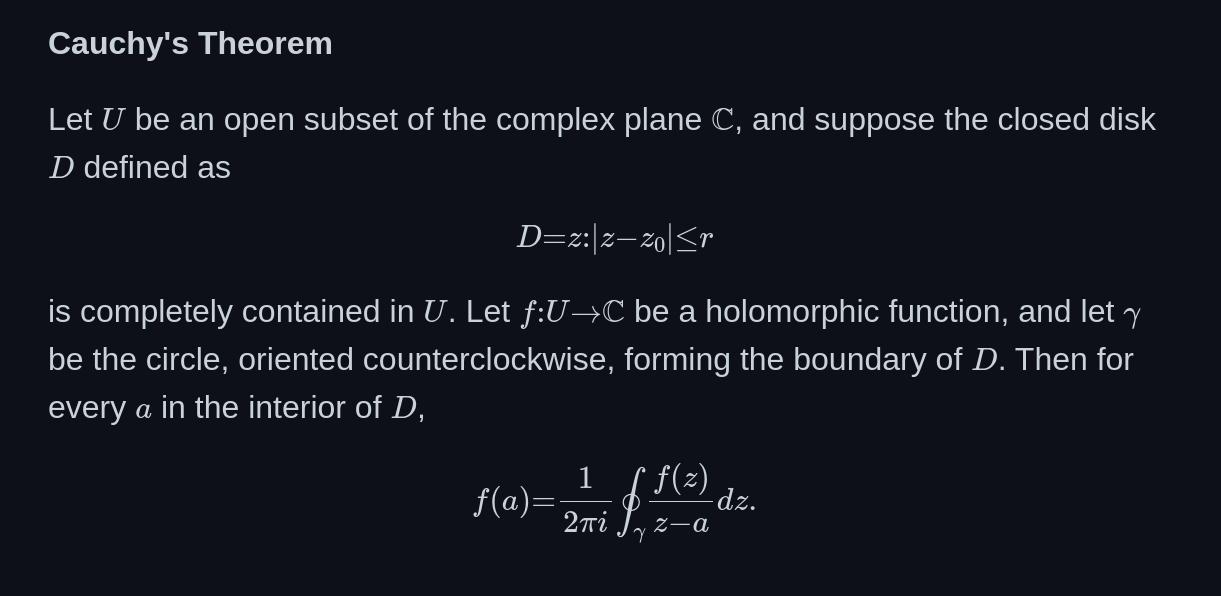You’ll notice that not everything is working here: • The curly brackets are missing in the first indented code block. (bug report here) • The math font is a really small, particularly the every a in towards the end. • The spacing between the math characters is wrong. (bug report here) (fixed now) • The syntax highlighting in the above code block is off. Apart from that: Great! This works in README.mds, issues, discussions, including the Preview tab. Note: In an earlier version, the article said that \newcommand would work. Apparently this has been removed shortly after release. See here for a bug report. ## The Bad ### The syntax Getting only one of Markdown and LaTeX rendering right is a big challenge. Mixing them together gets you in all kinds of trouble. What I think GitHub does now is the following: 1. Render the entire page as plain Markdown, produce the HTML. ($-$$is treated like text.) 2. Look for $$...$$ without intermediate HTML tags and render as math. 3. Do the same for ... pairs. This logic has fundamental issues. #### Competing Markdown and math renderers As part of step 1, the Markdown is “sanitized”. This means the removal of HTML tags unless they are allowed, or the removal of \ unless they are right before a letter (except in code blocks). This also affects everything in math, meaning that, e.g., • \{n\in\mathbb{N}:\: n \text{even}\}  gets “sanitized” to {n\in\mathbb{N}:: n \text{even}}  which as math renders like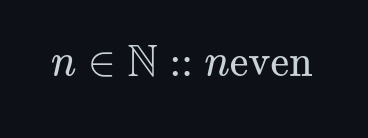• markdown$$
• a =
• b $$ Doesn’t get rendered as math because Markdown recognizes a list structure and transforms it to HTML.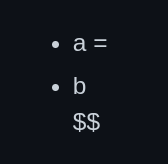• $$
a+b
<ul><li>c</li></ul>
$$ No math for the same reason: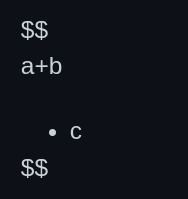• $$
a + b
<img/>
$$ This doesn’t render at all. • $$
a < b > c
$$ This gets rendered as math (with bad kerning, see below)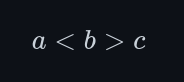This though $$
a <b > c

### It doesn’t work everywhere

Edit (May 28, 2022): Most of this has been fixed now.

Not everything works. Most notably, there is no math support in

• lists,
• tables,

yet.

- $E = mc^2$
- $a^2 + b^2 = c^2$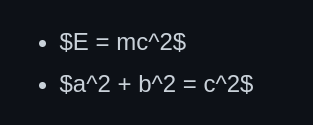(See here for a bug report.)

Getting math in lists etc. right is hard because of the syntax GitHub chose. With GitLab’s syntax, math works wherever Markdown code blocks work.

### MathJax vs KaTeX

Then there is their choice of rendering engine, MathJax. Historically, it’s the first engine that could seriously render math in web pages, and is used in many places, e.g., math.stackexchange. If you look just a little closer, though, you’ll find there’s at least one serious competitor: KaTeX. In terms of GitHub stars, it has become way more popular than MathJax: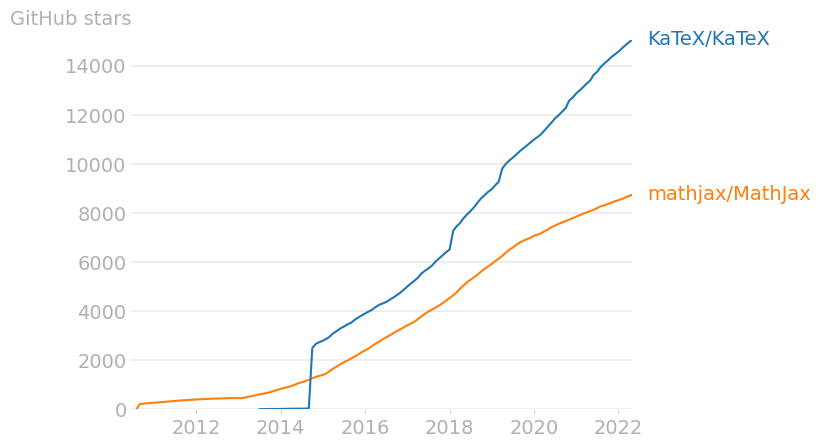Why is that? Having worked with both MathJax and KaTeX in xhub, I think the reasons for the popularity include

• its great community support
• It’s faster.
• It’s smaller/more modular (page load times!)
• You can copy-and-paste math
• Better font support

For one, with KaTeX I’ve never had problems as described in the Ugly section below.

Note: In a former version on this article, it was claimed that MathJax is barely maintained. This is not true.

## The Ugly

### Font sizes

MathJax’s default font MJXTEX-I and GitHub’s default text Helvetica have a different x-height/cap-height ratio. This means that, if you match the capitals in height, the small letters won’t match. This is what it looks like: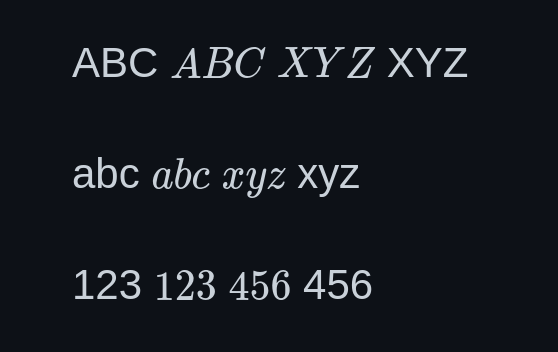Font enthusiasts will also notice that the text font is sans-serif while the math font has serifs – a no-go in serious typesetting, but forgivable on the web.

There’s probably not too much you can do here. Matching text and math fonts is a whole branch of science, see, e.g., here.

See the bug report here.

### Kerning

Edit (May 28, 2022): This has been fixed now.

Kerning is a typographist’s way of saying distance between letters. Compare the kerning in a = b between GitHub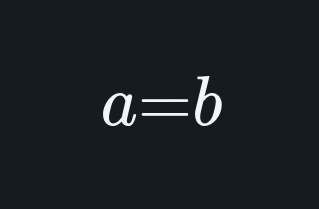and LaTeX: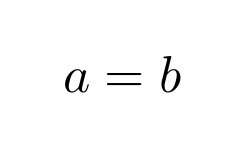Clearly, the GitHub kerning is off. This could perhaps be a font issue, or an issue related to MathJax. (See the bug report here.)

## Concluding thoughts

The reason why I’m so excited about this feature is that, in combination with version control and the issues/discussions capabilities in GitHub, I can see tectonic changes in how we’re publishing science. At last, science can really reap the benefits of a connected internet by moving away from static PDFs to living, breathing repositories which render like PDFs and provide a central place where one can actually talk about the article. – And fix bugs!

I’m less enthusiastic about some of the engineering decisions in developing this product though. The choice of MathJax over KaTeX confuses me. Since that is more or less a drop-in replacement, perhaps that’s a change that can be made at some point.

A more serious issue is GitHub’s approach of (not) trying to consolidate math and Markdown syntax. Something is working now, but knowing how hard it is to write a parser I’m pessimistic about how quickly bugs can be fixed and features added. The fact that math support is only on the top level of the document speaks for itself.

For these reasons, I would still recommend xhub for math on GitHub. Because of its choice of syntax, it doesn’t have any of GitHub-math’s problems. Cauchy’s theorem is rendered like